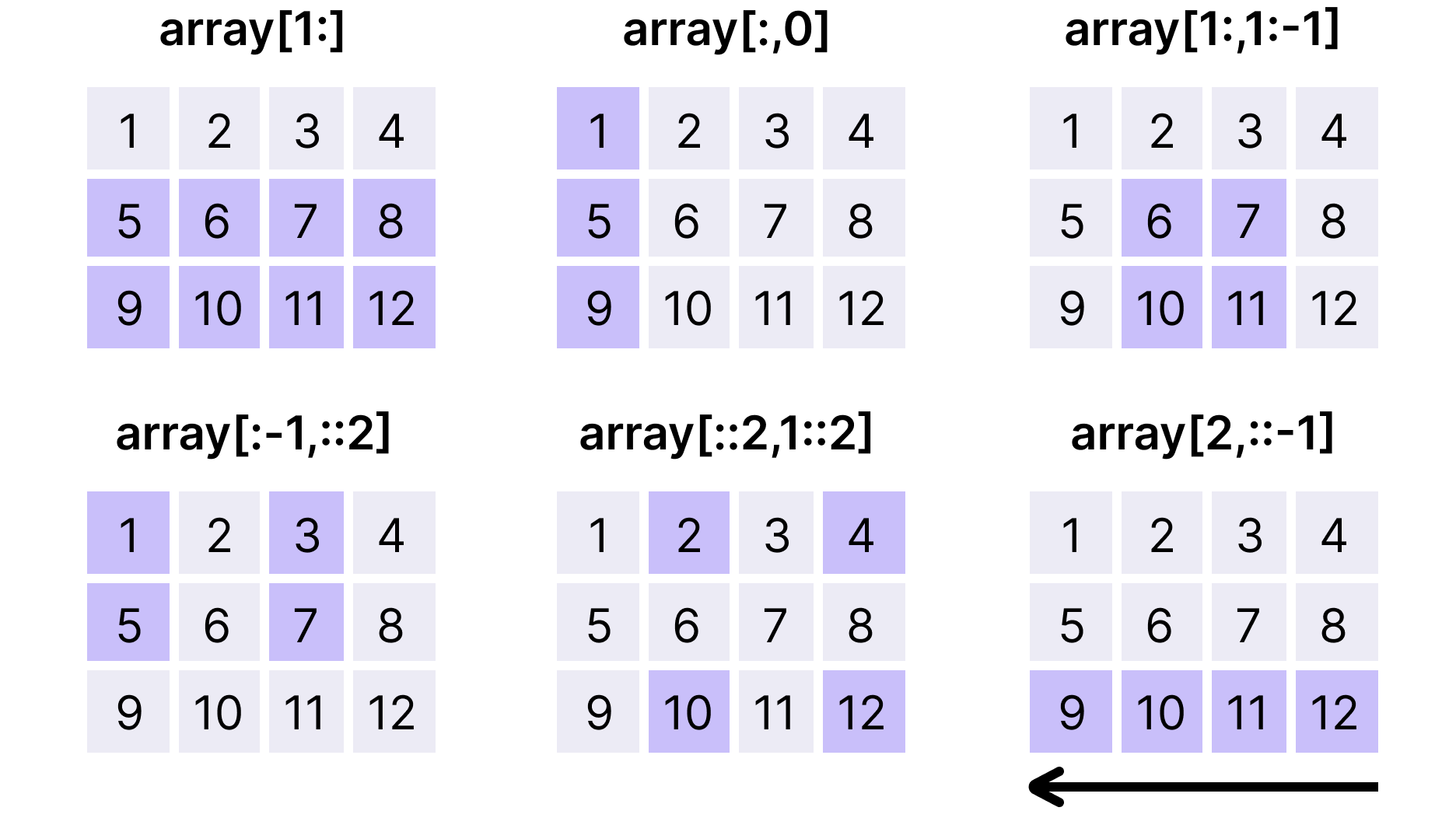Course Content

Ultimate NumPy

##Slicing

Slicing in Python stands for retrieving elements from a certain index to another index in a sequence. In this chapter, however, we will focus on slicing in NumPy arrays.

### Slicing in 1D Arrays

General syntax for slicing in 1D arrays is the following: `array[start:end:step]`. Let’s have a more detailed look at it:

• `start` is the index from which to start slicing;
• `end` is the index at which the slicing ends (the index itself is not included);
• `step` specifies the increments between the indices (`1` by default).

Here is an example to clarify everything:### Omitting start, end and step

As you can see, we can often omit the `start`, `end`, `step` or even all of them at the same time. `step`, for example, can be omitted when we want it to be equal to `1`. `start` and `end` can be omitted in the following scenarios:

1. Omitting `start`:
• slicing from the first element (`step` is positive);
• slicing from the last element (`step` is negative).
2. Omitting `end`:
• slicing to the last element inclusive (`step` is positive);
• slicing to the first element inclusive (`step` is negative).

### Slicing in 2D Arrays

Slicing in 2D and higher dimensional arrays works in a similar way as it does in 1D arrays. However, in 2D arrays there are already two axes.

If we want to perform slicing only on axis 0 to retrieve 1D arrays, then the syntax remains the same: `array[start:end:step]`. In case we want to perform slicing on the elements of these 1D arrays (axis 1), then the syntax is the following: `array[start:end:step, start:end:step]`. Basically, in this case the number of slices is equal to the number of dimensions of an array.

Moreover, we can use slicing for one axis and basic indexing for the other axis. Let’s have a look at an example with 2D slicing:The purple squares are the elements retrieved from slicing. Here is the code for this example:1. Create a slice of `array_1d` with every second element starting from the second element to the end and store it in `slice_1d` (use positive index for `start` and don't specify `end`).
2. Create a slice of `array_2d` with the two last elements of the first row (first 1D array) using basic indexing (positive indexing) and slicing (specify only positive `start`).